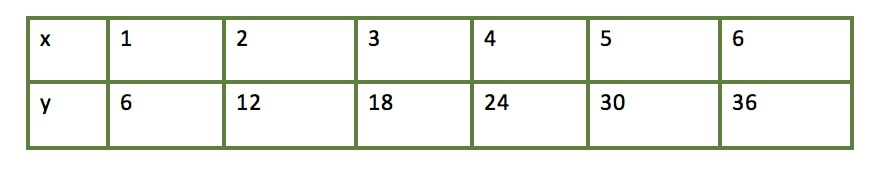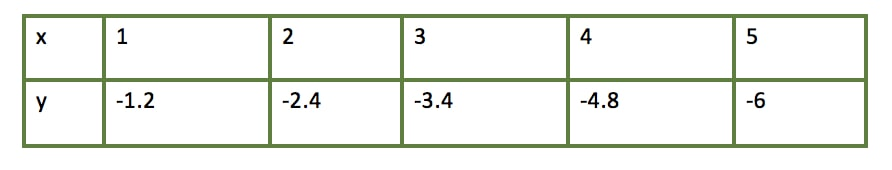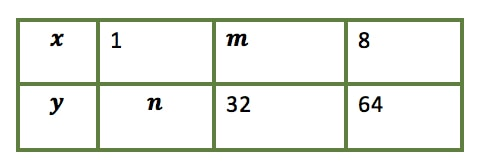# Direct variation

### Direct variation

#### Lessons

Direct Variation:
$y$ varies directly with $x$ with a constant variation of $k$

$y = kx$

where
$k \neq$ 0

• 1.
Identifying direct variations by table of values
Do the following tables of values represent direct variation?• 2.
Identifying direct variations
Which of the following represent direct variation?

A. $y$ = 2

B. $y$ = 3.1$x$

C. $y = - \frac{7x}{9}$

D. $xy$ = 191

E. $y$ = 8$x$ + 5

• 3.
Variable $y$ varies directly with $x$. Determine $m$ and $n$• 4.
If $a$ varies directly as $b$, and $b$ = 4 when $a$ = 16, find $a$ when $b = \frac{3}{2}$

• 5.
If the volume of an object varies directly as the cube of its height, and the volume equals 40$ft^{3}$ when the height is 2$ft$, find the object's volume when its height equals $\frac{1}{3} ft$

##### Do better in math today
6.
Direct and Inverse Variation
6.1
Direct variation